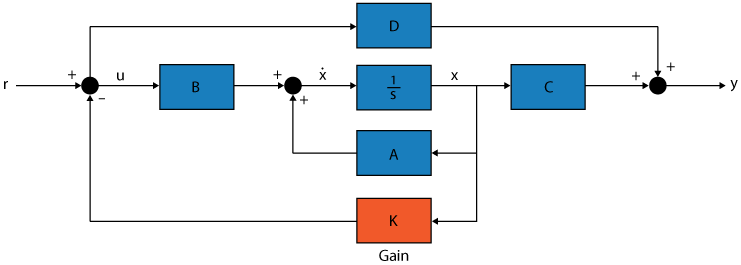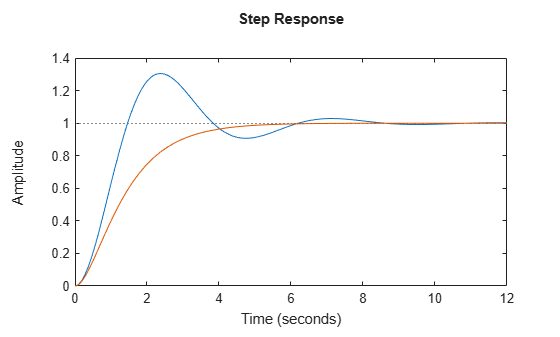# place

Pole placement design

## Syntax

``K = place(A,B,p)``
``[K,prec] = place(A,B,p)``

## Description

Pole placement is a method of calculating the optimum gain matrix used to assign closed-loop poles to specified locations, thereby ensuring system stability. Closed-loop pole locations have a direct impact on time response characteristics such as rise time, settling time, and transient oscillations. For more information, see Pole Placement.From the figure, consider a linear dynamic system in state-space form

`$\stackrel{˙}{x}=Ax+Bu$`

`$y=Cx+Du$`

For a given vector `p` of desired self-conjugate closed-loop pole locations, `place` computes a gain matrix `K` such that the state feedback u = –Kx places the poles at the locations `p`. In other words, the eigenvalues of ABK will match the entries of `p` (up to the ordering).

example

````K = place(A,B,p)` places the desired closed-loop poles `p` by computing a state-feedback gain matrix `K`. All the inputs of the plant are assumed to be control inputs. `place` also works for multi-input systems and is based on the algorithm from . This algorithm uses the extra degrees of freedom to find a solution that minimizes the sensitivity of the closed-loop poles to perturbations in A or B.```
````[K,prec] = place(A,B,p)` also returns `prec`, an accuracy estimate of how closely the eigenvalues of A – BK match the specified locations `p` (`prec` measures the number of accurate decimal digits in the actual closed-loop poles). A warning is issued if some nonzero closed-loop pole is more than 10% off from the desired location.```

## Examples

collapse all

For this example, consider a simple second-order system with the following state-space matrices:

`$A=\left[\begin{array}{cc}-1& -2\\ 1& 0\end{array}\right]\phantom{\rule{0.2777777777777778em}{0ex}}\phantom{\rule{0.2777777777777778em}{0ex}}\phantom{\rule{0.2777777777777778em}{0ex}}\phantom{\rule{0.2777777777777778em}{0ex}}B=\left[\begin{array}{c}2\\ 0\end{array}\right]\phantom{\rule{0.2777777777777778em}{0ex}}\phantom{\rule{0.2777777777777778em}{0ex}}\phantom{\rule{0.2777777777777778em}{0ex}}C=\left[\begin{array}{cc}0& 1\end{array}\right]\phantom{\rule{0.2777777777777778em}{0ex}}\phantom{\rule{0.2777777777777778em}{0ex}}\phantom{\rule{0.2777777777777778em}{0ex}}D=0$`

Input the matrices and create the state-space system.

```A = [-1,-2;1,0]; B = [2;0]; C = [0,1]; D = 0; sys = ss(A,B,C,D);```

Compute the open-loop poles and check the step response of the open-loop system.

`Pol = pole(sys)`
```Pol = 2×1 complex -0.5000 + 1.3229i -0.5000 - 1.3229i ```
```figure(1) step(sys) hold on;```Notice that there is an unstable pole, and the resultant system is underdamped. Hence, choose real poles in the left half of the complex-plane to remove oscillations.

`p = [-1,-2];`

Find the gain matrix `K` using pole placement and check the closed-loop poles of `syscl`.

```K = place(A,B,p); Acl = A-B*K; syscl = ss(Acl,B,C,D); Pcl = pole(syscl)```
```Pcl = 2×1 -2.0000 -1.0000 ```

Now, compare the step response of the closed-loop system.

```figure(1) step(syscl)```Hence, the closed-loop system obtained using pole placement is stable with good steady-state response.

Note that choosing poles that are further away from the imaginary axis achieves faster response time but lowers the steady-state gain of the system. For instance, consider using the poles `[-2,-3]` for the above system.

```p = [-2, -3]; K2 = place(A,B,p); syscl2 = ss(A-B*K2,B,C,D); figure(1); step(syscl2);````stepinfo(syscl)`
```ans = struct with fields: RiseTime: 2.5901 TransientTime: 4.6002 SettlingTime: 4.6002 SettlingMin: 0.9023 SettlingMax: 0.9992 Overshoot: 0 Undershoot: 0 Peak: 0.9992 PeakTime: 7.7827 ```
`stepinfo(syscl2)`
```ans = struct with fields: RiseTime: 1.4130 TransientTime: 2.4766 SettlingTime: 2.4766 SettlingMin: 0.3003 SettlingMax: 0.3331 Overshoot: 0 Undershoot: 0 Peak: 0.3331 PeakTime: 4.1216 ```

For this example, consider the pole locations `[-2e-13,-3e-4,-3e-3]`. Compute the precision of the actual poles.

```A = [4,2,1;0,-1,2;0,1e-8,1]; B = [1,2;3,1;1e-6,0]; p = [-2e-13,-3e-4,3e-3]; [~,prec] = place(A,B,p)```
```prec = 2 ```

A precision value of 2 is obtained indicating that the actual pole locations are precise up to 2 decimal places.

For this example, consider the following transfer function with complex-conjugate poles at $-2±2\mathit{i}$:

`$systf\left(s\right)=\frac{8}{{s}^{2}+4s+8}$`

Input the transfer function model. Then, convert it to state-space form since `place` uses the `A` and `B` matrices as input arguments.

```s = tf('s'); systf = 8/(s^2+4*s+2); sys = ss(systf);```

Next, compute the gain matrix `K` using the complex-conjugate poles.

```p = [-2+2i,-2-2i]; K = place(sys.A,sys.B,p)```
```K = 1×2 0 1.5000 ```

The values of the gain matrix are real since the poles are self-conjugate. The values of `K` would be complex if `p` did not contain self-conjugate poles.

Now, verify the step response of the closed-loop system.

```syscl = ss(sys.A-sys.B*K,sys.B,sys.C,sys.D); step(syscl)```For this example, consider the following SISO state-space model:

`$A=\left[\begin{array}{cc}-1& -0.75\\ 1& 0\end{array}\right]\phantom{\rule{0.2777777777777778em}{0ex}}\phantom{\rule{0.2777777777777778em}{0ex}}\phantom{\rule{0.2777777777777778em}{0ex}}\phantom{\rule{0.2777777777777778em}{0ex}}B=\left[\begin{array}{c}1\\ 0\end{array}\right]\phantom{\rule{0.2777777777777778em}{0ex}}\phantom{\rule{0.2777777777777778em}{0ex}}\phantom{\rule{0.2777777777777778em}{0ex}}\phantom{\rule{0.2777777777777778em}{0ex}}C=\left[\begin{array}{cc}1& 1\end{array}\right]\phantom{\rule{0.2777777777777778em}{0ex}}\phantom{\rule{0.2777777777777778em}{0ex}}\phantom{\rule{0.2777777777777778em}{0ex}}\phantom{\rule{0.2777777777777778em}{0ex}}D=0$`

Create the SISO state-space model defined by the following state-space matrices:

```A = [-1,-0.75;1,0]; B = [1;0]; C = [1,1]; D = 0; Plant = ss(A,B,C,D);```

Now, provide a pulse to the plant and simulate it using `lsim`. Plot the output.

```N = 250; t = linspace(0,25,N); u = [ones(N/2,1); zeros(N/2,1)]; x0 = [1;2]; [y,t,x] = lsim(Plant,u,t,x0); figure plot(t,y); title('Output');```For this example, assume that all the state variables cannot be measured and only the output is measured. Hence, design an observer with this measurement. Use `place` to compute the estimator gain by transposing the `A` matrix and substituting `C'` for matrix `B`. For this instance, select the desired pole locations at `-2` and `-3`.

`L = place(A',C',[-2,-3])';`

Use the estimator gain to substitute the state matrices using the principle of duality/separation and create the estimated state-space model.

```At = A-L*C; Bt = [B,L]; Ct = [C;eye(2)]; sysObserver = ss(At,Bt,Ct,0);```

Simulate the time response of the system using the same pulse input.

```[observerOutput,t] = lsim(sysObserver,[u,y],t); yHat = observerOutput(:,1); xHat = observerOutput(:,[2 3]);```

Compare the response of the actual system and the estimated system.

```figure; plot(t,x); hold on; plot(t,xHat,'--'); legend('x_1','x_2','xHat_1','xHat_2') title('Comparison - Actual vs. Estimated');```## Input Arguments

collapse all

State matrix, specified as an `Nx`-by-`Nx` matrix where, `Nx` is the number of states.

Input-to-state matrix, specified as an `Nx`-by-`Nu` matrix where, `Nx` is the number of states and `Nu` is the number of inputs.

Closed-loop pole locations, specified as a vector of length `Nx` where, `Nx` is the number of states. In other words, the length of `p` must match the row size of `A`. Closed-loop pole locations have a direct impact on time response characteristics such as rise time, settling time, and transient oscillations. For an example on selecting poles, see Pole Placement Design for Second-Order System.

`place` returns an error if some poles in `p` have multiplicity greater than `rank(B)`.

In high-order problems, some choices of pole locations result in very large gains. The sensitivity problems attached with large gains suggest caution in the use of pole placement techniques. See  for results from numerical testing.

## Output Arguments

collapse all

Optimum gain or full-state feedback gain, returned as an `Ny`-by-`Nx` matrix where, `Nx` is the number of states and `Ny` is the number of outputs. `place` computes a gain matrix `K` such that the state feedback u = –Kx places the closed-loop poles at the locations `p`.

When the matrices `A` and `B` are real, `K` is

• real when `p` is self-conjugate.

• complex when the pole locations are not complex-conjugates.

Accuracy estimate of the assigned poles, returned as a scalar. `prec` measures the number of accurate decimal digits in the actual closed-loop poles in contrast to the pole locations specified in `p`.

## Tips

• You can use `place` for estimator gain selection by transposing the `A` matrix and substituting `C'` for matrix `B` as follows, as shown in Pole Placement Observer Design. You can use the resultant estimator gain for state estimator workflows using `estim`.

 Kautsky, J., N.K. Nichols, and P. Van Dooren, "Robust Pole Assignment in Linear State Feedback," International Journal of Control, 41 (1985), pp. 1129-1155.

 Laub, A.J. and M. Wette, Algorithms and Software for Pole Assignment and Observers, UCRL-15646 Rev. 1, EE Dept., Univ. of Calif., Santa Barbara, CA, Sept. 1984.